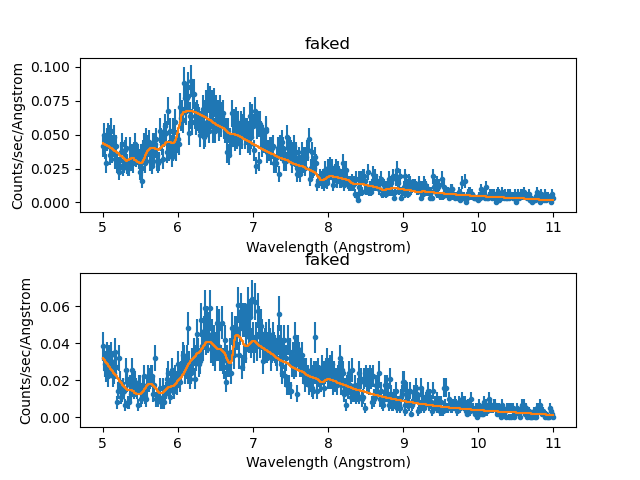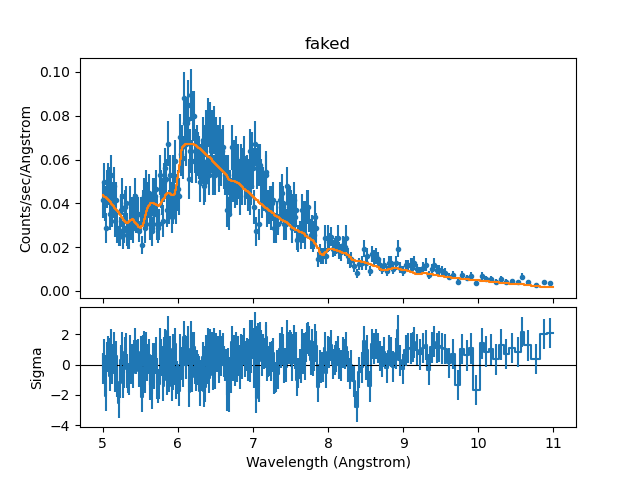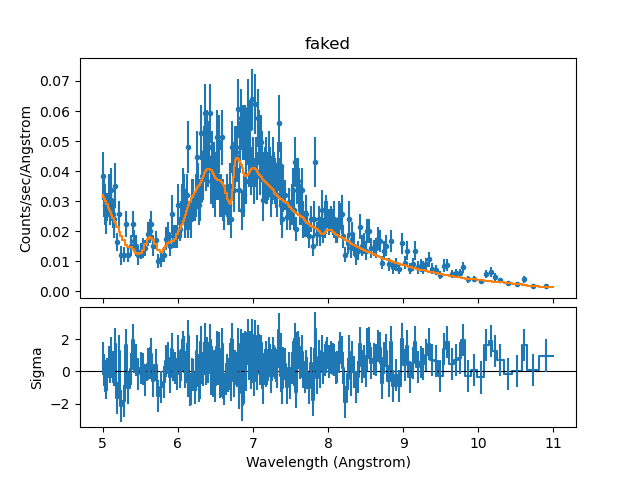# Simulating Chandra ACIS-S LETG Spectra with Sherpa## Overview

#### Synopsis:

This thread illustrates the use of the Sherpa fake_pha command to simulate a spectrum of a point source as would be observed with a different Chandra instrument configuration than that used to produce the user's existing data and source model. We simulate ACIS-S/LETG ±1 LEG spectral orders using a source model expression tailored to the ±1 HEG and MEG orders of a real ACIS-S/HETG data set.

If you do not have experience with simulating X-ray spectral data in Sherpa, you may wish to follow the "step-by-step" example in the introductory simulation thread, before attempting the sample analysis in this thread.

Last Update: 13 Dec 2022 - updated for Cycle 25 and CIAO 4.15, no new content.

## Contents

In order to simulate a Chandra ACIS-S/LETG spectrum with Sherpa, we must define an instrument response with the appropriate ARF (ancillary response function) and RMF (redistribution matrix function) files. These files may be downloaded from the Chandra Proposal Planning page of the CalDB (Calibration Database) website, where sample RMFs and corresponding ARFs positioned at the aimpoint of the ACIS-S array (and at selected off-axis points) are available.

In this thread, we use the files aciss_leg1_cy25.grmf, aciss_leg-1_cy25.grmf, aciss_leg1_cy25.garf, and aciss_leg-1_cy25.garf, positioned at the default pointing for ACIS-S.

The Sherpa fake_pha command calculates a synthetic 1-D spectral data set based on a defined instrument response and source model expression. For details on the functionality of this command, with examples of its usage, see the threads "Simulating 1-D Data: the Sherpa FAKE_PHA Command" and "Simulating Chandra ACIS-S Spectra with Sherpa"

Sample ObsID used: 459 (HETG/ACIS-S, 3C 273)

The ACIS-S/HETG data files used to establish the appropriate source model expression for the simulated data were created by following several of the CIAO Grating threads:

The following files were loaded into Sherpa and analyzed according to the detailed steps shown here, in order to establish the source model expression to be used with fake_pha:

```spectra:
459_heg_m1_bin10.pha
459_heg_p1_bin10.pha
459_meg_m1_bin10.pha
459_meg_p1_bin10.pha

gARFs:
459_heg_m1.arf
459_heg_p1.arf
459_meg_m1.arf
459_meg_p1.arf

```

The spectra used in the fitting session were binned by a factor of 10. The data files are available in sherpa.tar.gz, as explained in the Sherpa Getting Started thread.

## Defining the Instrument Response for the Simulation

Armed with a source model expression resulting from a satisfactory fit to the ACIS-S/HETG data, we may begin the simulation process by establishing the instrument response corresponding to the default pointing of the ACIS-S detector, using the ACIS-S/LETG grating ARF and RMF files retrieved from the CalDB website:

```sherpa> arf1 = unpack_arf("aciss_leg1_cy25.garf")
sherpa> arf2 = unpack_arf("aciss_leg-1_cy25.garf")

sherpa> print(arf1)
name     = aciss_leg1_cy25.garf
energ_lo = Float64
energ_hi = Float64
specresp = Float64
bin_lo   = Float64
bin_hi   = Float64
exposure = 28398.454681545
ethresh  = 1e-10

sherpa> print(arf2)
name     = aciss_leg-1_cy25.garf
energ_lo = Float64
energ_hi = Float64
specresp = Float64
bin_lo   = Float64
bin_hi   = Float64
exposure = 28398.489680535
ethresh  = 1e-10

sherpa> rmf1 = unpack_rmf("aciss_leg1_cy25.grmf")
sherpa> rmf2 = unpack_rmf("aciss_leg-1_cy25.grmf")

sherpa> print(rmf1)
name     = aciss_leg1_cy25.grmf
energ_lo = Float64
energ_hi = Float64
n_grp    = UInt64
f_chan   = UInt32
n_chan   = UInt32
matrix   = Float64
e_min    = Float64
e_max    = Float64
detchans = 8192
offset   = 1
ethresh  = 1e-10

sherpa> print(rmf2)
name     = aciss_leg-1_cy25.grmf
energ_lo = Float64
energ_hi = Float64
n_grp    = UInt64
f_chan   = UInt32
n_chan   = UInt32
matrix   = Float64
e_min    = Float64
e_max    = Float64
detchans = 8192
offset   = 1
ethresh  = 1e-10
```

Here, the ARF and RMF data are loaded and assigned to a set of variables with the unpack_arf and unpack_rmf commands. These variables will be used to assign the instrument response to the faked data sets we will create in the next section, "Running the Simulation with fake_pha."

## Establishing the Source Model Expression for the Simulation

The fake_pha command has four required arguments: data set ID, ARF, RMF, and exposure time. In this thread, we simulate a LETG spectrum with the source model parameter values resulting from a fit to a HETG grating spectrum. The detailed steps taken to establish this model can be found here, and include:

• defining the instrument response for the real data
• filtering the data
• defining the source and background models
• examining method & statistic settings
• fitting
• examining fit results

This saved Sherpa session, including all defined data sets, models, and instrument responses, may be restored with the Sherpa restore command, as follows:

```sherpa> restore("acis_hetg_fit_results.save")
```

If restore fails to load the state file, due to version incompatibility, the source model defined in the file can be loaded into Sherpa:

```sherpa> set_analysis("wave")
sherpa> set_source(1, atten.abs1 * bpl1d.bpow1)

sherpa> abs1.hcol = 1.81e+20
sherpa> abs1.heiRatio = 0.1
sherpa> abs1.heiiRatio = 0.01
sherpa> bpow1.gamma1 = 0.424711
sherpa> bpow1.gamma2 = 0.0496577
sherpa> bpow1.eb = 10.62
sherpa> bpow1.ref = 1
sherpa> bpow1.ampl = 0.00548927

sherpa> freeze(abs1)
```

Since we want to run the simulation using the source model tailored to the ACIS-S/HETG data, we use the set_source command to assign this source model expression to each of the LEG orders, as well as name the corresponding faked data sets which will be produced in our simulation.

The following steps assume that the ACIS-S/HETG spectral orders correspond to data set IDs 1 through 4 in the saved state file.

```sherpa> show_source(1)
Model: 1
(atten.abs1 * bpl1d.bpow1)
Param        Type          Value          Min          Max      Units
-----        ----          -----          ---          ---      -----
abs1.hcol    frozen     1.81e+20        1e+17        1e+24
abs1.heiRatio frozen          0.1            0            1
abs1.heiiRatio frozen         0.01            0            1
bpow1.gamma1 thawed     0.424711          -10           10
bpow1.gamma2 thawed    0.0496577          -10           10
bpow1.eb     thawed        10.62            0         1000
bpow1.ref    frozen            1 -3.40282e+38  3.40282e+38
bpow1.ampl   thawed   0.00548927        1e-20  3.40282e+38

sherpa> set_source("faked_leg1", get_source(1))
sherpa> set_source("faked_leg-1", get_source(1))
```

## Running the Simulation with fake_pha

Simulating the Chandra ACIS-S/LETG spectra means taking the model expression defined for the ACIS-S/HETG data, folding it through the Chandra ACIS-S/LETG response, and applying Poisson noise to the counts predicted by the model. The simulation is run with fake_pha, which has four required arguments: data set ID, ARF, RMF, and exposure time. In this thread, we will simulate ACIS-S/LETG spectra using the gARF and gRMF files downloaded from the CalDB website, a source model tailored to the ACIS-S/HETG data, and an exposure time of 50 ks.

We are now ready to run the simulations with fake_pha:

```sherpa> fake_pha("faked_leg1", arf1, rmf1, exposure=50000)

sherpa> fake_pha("faked_leg-1", arf2, rmf2, exposure=50000)
```

These commands associate the data IDs "faked_leg1" and "faked_leg-1" with simulated source data sets for the +1 and -1 ACIS-S/LETG orders, respectively, based on the assumed exposure time, instrument responses, and source model expression; Poisson noise is added to the modeled data.

Note that we could have said the following instead:

```sherpa> fake_pha("faked_leg1", arf="aciss_leg1_cy25.garf", rmf="aciss_leg1_cy25.grmf", exposure=50000)
sherpa> fake_pha("faked_leg-1", arf="aciss_leg-1_cy25.garf", rmf="aciss_leg-1_cy25.grmf", exposure=50000)
```

For detailed information on the available options for setting the 'arf' and 'rmf' arguments of fake_pha, refer to the fake_pha ahelp file.

We may inspect some basic properties of the new data sets with the show_data command; e.g.,:

```sherpa> show_data("faked_leg1")
Data Set: faked_leg1
Filter: 0.1199-12.3984 Energy (keV)
Noticed Channels: 1-8192
name           = faked
channel        = Int64
counts         = Float64
staterror      = None
syserror       = None
bin_lo         = None
bin_hi         = None
grouping       = None
quality        = None
exposure       = 50000
backscal       = None
areascal       = None
grouped        = False
subtracted     = False
units          = energy
rate           = True
plot_fac       = 0
response_ids   = 
background_ids = []

RMF Data Set: faked_leg1:1
name     = aciss_leg1_cy25.grmf
energ_lo = Float64
energ_hi = Float64
n_grp    = UInt64
f_chan   = UInt32
n_chan   = UInt32
matrix   = Float64
e_min    = Float64
e_max    = Float64
detchans = 8192
offset   = 1
ethresh  = 1e-10

ARF Data Set: faked_leg1:1
name     = aciss_leg1_cy25.garf
energ_lo = Float64
energ_hi = Float64
specresp = Float64
bin_lo   = Float64
bin_hi   = Float64
exposure = 28398.454681545
ethresh  = 1e-10

sherpa> show_data("faked_leg-1")
Data Set: faked_leg-1
Filter: 0.1199-12.3984 Energy (keV)
Noticed Channels: 1-8192
name           = faked
channel        = Int64
counts         = Float64
staterror      = None
syserror       = None
bin_lo         = None
bin_hi         = None
grouping       = None
quality        = None
exposure       = 50000
backscal       = None
areascal       = None
grouped        = False
subtracted     = False
units          = energy
rate           = True
plot_fac       = 0
response_ids   = 
background_ids = []

RMF Data Set: faked_leg-1:1
name     = aciss_leg-1_cy25.grmf
energ_lo = Float64
energ_hi = Float64
n_grp    = UInt64
f_chan   = UInt32
n_chan   = UInt32
matrix   = Float64
e_min    = Float64
e_max    = Float64
detchans = 8192
offset   = 1
ethresh  = 1e-10

ARF Data Set: faked_leg-1:1
name     = aciss_leg-1_cy25.garf
energ_lo = Float64
energ_hi = Float64
specresp = Float64
bin_lo   = Float64
bin_hi   = Float64
exposure = 28398.489680535
ethresh  = 1e-10
```

Had we not applied a previously defined, properly normalized source model expression to the faked data, we would have had to re-normalize the simulated data to match the flux (or counts) required by the real source data. This process is illustrated in the threads "Simulating Chandra ACIS-S Spectra with Sherpa" and "Simulating 1-D Data: the Sherpa FAKE_PHA Command."

## Writing the Simulated Data to Output Files

We may use the save_pha command to write the simulated data as a PHA file, with a header containing the exposure time value and paths to the ARF and RMF files:

```sherpa>save_pha("faked_leg1","simulation1.pha")
sherpa>save_pha("faked_leg-1","simulation-1.pha")
```

There is also an option to save the data to an ASCII file:

```sherpa>save_pha("faked_leg1","simulation1.dat", ascii=True)
sherpa>save_pha("faked_leg-1","simulation-1.dat", ascii=True)
```

## Fitting the Simulated Data

The simulated data set may be filtered and fit as any other data set in Sherpa.

```sherpa> set_analysis("wave")
sherpa> ignore()
dataset faked_leg-1: 1:103.4 Wavelength (Angstrom) -> no data
dataset faked_leg1: 1:103.4 Wavelength (Angstrom) -> no data

sherpa> notice(5., 11.)
dataset faked_leg-1: no data -> 5:11 Wavelength (Angstrom)
dataset faked_leg1: no data -> 5:11 Wavelength (Angstrom)

sherpa> show_filter()
Data Set Filter: faked_leg-1
5.0000-11.0000 Wavelength (Angstrom)

Data Set Filter: faked_leg1
5.0000-11.0000 Wavelength (Angstrom)
```

Then, we can fit the simulated data with the source model expression we used to create it and restrict the wavelength range that the power-law break point is explored:

```sherpa> set_method("neldermead")
sherpa> set_stat("chi2xspecvar")
sherpa> bpow1.eb.max = 11
sherpa> bpow1.eb.min = 5

sherpa> fit("faked_leg1", "faked_leg-1")
Datasets              = 'faked_leg1', 'faked_leg-1'
Statistic             = chi2xspecvar
Initial fit statistic = 31424.4
Final fit statistic   = 1042.09 at function evaluation 816
Data points           = 960
Degrees of freedom    = 956
Probability [Q-value] = 0.0269443
Reduced statistic     = 1.09005
Change in statistic   = 30382.3
bpow1.gamma1   1.30872
bpow1.gamma2   2.10441
bpow1.eb       6.97175
bpow1.ampl     0.0137372

sherpa> plot("fit","faked_leg1","fit","faked_leg-1")
```

The resulting plot is shown in Figure 1.

### Figure 1: Plot of fit to simulated ACIS-S/LETG source spectra### Figure 1: Plot of fit to simulated ACIS-S/LETG source spectra

Plot of fit to simulated ACIS-S/LETG ±1 spectral orders, using a source model tailored to a real ACIS-S/HETG data set.

Next, we examine the quality of the fit with the confidence method (conf), and print the fit and confidence results with the show_fit and get_conf_results commands, respectively.

```sherpa> conf("faked_leg1", "faked_leg-1")
bpow1.gamma2 lower bound:	-0.100914
bpow1.gamma2 upper bound:	0.105381
bpow1.eb lower bound:	-0.141073
bpow1.eb upper bound:	0.157618
bpow1.gamma1 lower bound:	-0.112896
bpow1.gamma1 upper bound:	0.109072
bpow1.ampl lower bound:	-0.00255392
bpow1.ampl upper bound:	0.00304823
Datasets              = 'faked_leg1', 'faked_leg-1'
Confidence Method     = confidence
Iterative Fit Method  = None
Statistic             = chi2xspecvar
confidence 1-sigma (68.2689%) bounds:
Param            Best-Fit  Lower Bound  Upper Bound
-----            --------  -----------  -----------
bpow1.gamma1      1.30872    -0.112896     0.109072
bpow1.gamma2      2.10441    -0.100914     0.105381
bpow1.eb          6.97175    -0.141073     0.157618
bpow1.ampl      0.0137372  -0.00255392   0.00304823

sherpa> show_fit()
name         = simplex
ftol         = 1.1920928955078125e-07
maxfev       = None
initsimplex  = 0
finalsimplex = 9
step         = None
verbose      = 0
reflect      = True

Statistic: Chi2XspecVar
Chi Squared with data variance (XSPEC style).

The variance in each bin is estimated from the data value in that
bin.

The calculation of the variance is the same as `Chi2DataVar`
except that if the number of counts in a bin is less than 1
then the variance for that bin is set to 1.

--------
Chi2DataVar, Chi2Gehrels, Chi2ModVar

Fit:Datasets              = 'faked_leg1', 'faked_leg-1'
Statistic             = chi2xspecvar
Initial fit statistic = 31424.4
Final fit statistic   = 1042.09 at function evaluation 816
Data points           = 960
Degrees of freedom    = 956
Probability [Q-value] = 0.0269443
Reduced statistic     = 1.09005
Change in statistic   = 30382.3
bpow1.gamma1   1.30872
bpow1.gamma2   2.10441
bpow1.eb       6.97175
bpow1.ampl     0.0137372   )

sherpa> print(get_conf_results())
datasets    = ('faked_leg1', 'faked_leg-1')
methodname  = confidence
iterfitname = none
statname    = chi2xspecvar
sigma       = 1
percent     = 68.26894921370858
parnames    = ('bpow1.gamma1', 'bpow1.gamma2', 'bpow1.eb', 'bpow1.ampl')
parvals     = (1.3087226286343174, 2.1044061775565943, 6.971748448295852, 0.013737218447832955)
parmins     = (-0.11289621593988297, -0.10091380623038049, -0.1410733795542889, -0.0025539244495105754)
parmaxes    = (0.10907213741866295, 0.105380538929154, 0.15761833385712354, 0.003048232407480365)
nfits       = 56
```

We can group the data so that each bin contains a specified minimum number of counts, and then change the fit statistic to something more suitable to calculate the errors. Finally, we can view the results of the new fit with the plot_fit_delchi command:

```sherpa> group_counts("faked_leg1", 15)
sherpa> group_counts("faked_leg-1", 15)
sherpa> show_filter()
Data Set Filter: faked_leg-1
5.0000-11.0000 Wavelength (Angstrom)

Data Set Filter: faked_leg1
5.0000-11.0500 Wavelength (Angstrom)

sherpa> plot_fit_delchi("faked_leg1")
sherpa> plot_fit_delchi("faked_leg-1")
```

The new fits to the grouped, simulated source spectra, along with the residuals divided by the uncertainties, are shown in Figure 2 and Figure 3.

### Figure 2: Plot of fit to simulated ACIS-S/LETG +1 order spectrum, with residuals### Figure 3: Plot of fit to simulated ACIS-S/LETG -1 order spectrum, with residuals### Figure 3: Plot of fit to simulated ACIS-S/LETG -1 order spectrum, with residuals

The plots may be saved as PostScript files accordingly:

```sherpa> plot_fit_delchi("faked_leg1")
sherpa> plt.savefig("sim_fit_p1.ps")

sherpa> plot_fit_delchi("faked_leg-1")
sherpa> plt.savefig("sim_fit_m1.ps")
```

## Scripting It

The file fit.py is a Python script which performs the primary commands used above; it can be executed by typing %run -i fit.py on the Sherpa command line.

The Sherpa script command may be used to save everything typed on the command line in a Sherpa session:

```sherpa> script(filename="sherpa.log", clobber=False)
```

(Note that restoring a Sherpa session from such a file could be problematic since it may include syntax errors, unwanted fitting trials, et cetera.)

## History

 02 Apr 2009 Created for CIAO/Sherpa 4.1 28 Apr 2009 replaced use of atten model with Sherpa user model "atten_wave"; new script command is available with CIAO 4.1.2 13 Jan 2010 updated for CIAO 4.2; new calibration response files used in simulation 13 Jul 2010 updated for CIAO 4.2 Sherpa v2: removal of S-Lang version of thread. 15 Dec 2011 reviewed for CIAO 4.4: a work-around for a save_pha bug was added; response files used in simulations were updated for Chandra proposal cycle 13 13 Dec 2012 reviewed for CIAO 4.5: group commands no longer clear the existing data filter 12 Dec 2013 reviewed for CIAO 4.6: fixed typos, updated calibration files, show restore warning messages. 03 Mar 2014 updated for Chandra proposal cycle 16 30 Jan 2015 updated for Chandra proposal cycle 17 15 Dec 2015 updated for Chandra proposal cycle 18 06 Dec 2016 updated for Chandra proposal cycle 19; include defining source model found in the restored state file, that would fail to load in newer versions of Sherpa. 24 Apr 2018 updated for CIAO 4.10, no new content. 19 Mar 2019 updated for CIAO 4.11, fits revised. 11 Dec 2019 Updated for CIAO 4.12 by replacing print_window calls with the equivalent Matplotlib command plt.savefig. 18 Mar 2022 updated for CIAO 4.14 and CY 24, simulations done in wavelength-space and fits revised, changing to 'chi2xspec' statistics since many bins in synthetic spectra have zero counts and zero uncertainty, which is incompatible with 'chi2datavar'. 13 Dec 2022 updated for Cycle 25 and CIAO 4.15, no new content.